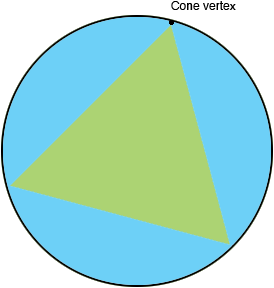SEARCH HOMEMath Central Quandaries & QueriesQuestion from joel, a student: how can I find the radius and the height of a cone INSCRIBED in a sphere, given the sphere having a radius of 6? ( note: the diameter of the cone is equal to its slanted height).Hi Joel,

I can help get you started.

Slice the sphere by a plane that passes through the centre of the sphere and the vertex of the cone. In the resulting plane figure join the points where the slicing plane meets the base circle of the cone.Since the diameter of the base of the cone and the slant height are equal the resulting triangle is equilateral.

PennyMath Central is supported by the University of Regina and The Pacific Institute for the Mathematical Sciences.